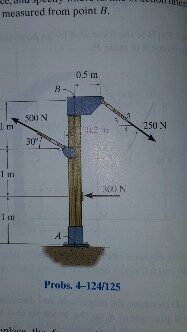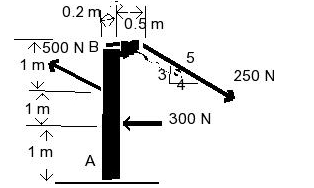# Problem: Replace the force system acting on the post by a resultant force and specify where its line of action intersects the post AB measured from point A.

###### FREE Expert Solution

Torque:

$\overline{){\mathbit{\tau }}{\mathbf{=}}{\mathbit{r}}{\mathbit{F}}}$

We'll determine the resultant force from the summation of Fx and Fy components.

Fx = Fcosθ

ΣFx = 250cosθ - 300 - 500cosθ = 250(4/5) - 300 - 500cos30 = -533N

Fy = Fsinθ

87% (423 ratings)###### Problem Details

Replace the force system acting on the post by a resultant force and specify where its line of action intersects the post AB measured from point A.